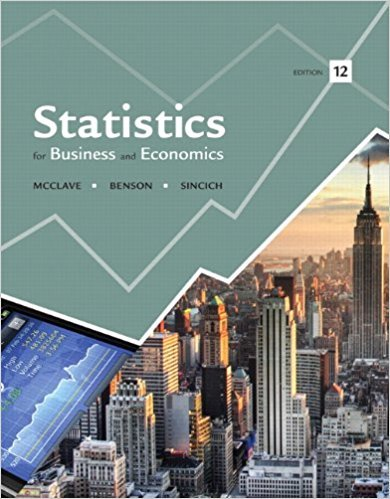×
Get Full Access to Statistics For Business And Economics - 12 Edition - Chapter 6 - Problem 90e
Get Full Access to Statistics For Business And Economics - 12 Edition - Chapter 6 - Problem 90e

×

# Invoice errors in a billing system. In a study of invoiceISBN: 9780321826237 51

## Solution for problem 90E Chapter 6

Statistics for Business and Economics | 12th Edition

• Textbook Solutions
• 2901 Step-by-step solutions solved by professors and subject experts
• Get 24/7 help from StudySoup virtual teaching assistantsStatistics for Business and Economics | 12th Edition

4 5 1 277 Reviews
28
1
Problem 90E

Problem 90E

Invoice errors in a billing system. In a study of invoice errors in a company’s new billing system, an auditor randomly sampled 35 invoices produced by the new system and recorded actual amount (A), invoice amount (I), and the difference (or error), x = (A - I). The results were= \$1 and s = +124. At the time that the sample was drawn, the new system had produced 1,500 invoices. Use this information to find an approximate 95% confidence interval for the true mean error per invoice of the new system. Interpret the result.

Step-by-Step Solution:

Solution :

Step 1 of 1:

The given problem explain about invoice errors in a billing system.

Let N denotes the population size is 1500 invoices.

Let n denotes the sample size is 35 invoices.

Letdenotes the sample mean of invoices is \$1 and

Let s denotes the standard deviation of the invoices is \$124.

Our goal is:

We need to find an approximate 95% confidence interval for the true mean error per invoice of the new system.

Now we have to find an approximate 95% confidence interval for the true mean error per invoice of the new system.

Now we have to find the approximate 95% confidence interval.

Then the estimated standard error isWe know that n, N, and s.20.7137

Therefore, the estimated standard error is 20.7137.

Then the approximate 95% confidence interval isWe know that= 1 and= 20.7137.=== (1-41.43, 1+41.43)

= (-40.43, 42.43)

Hence a 95% confidence interval for the true mean error per invoice of the new system is between -\$40.43 and \$42.43.

Step 2 of 1

##### ISBN: 9780321826237

This full solution covers the following key subjects: system, new, invoice, Billing, produced. This expansive textbook survival guide covers 15 chapters, and 1631 solutions. The answer to “Invoice errors in a billing system. In a study of invoice errors in a company’s new billing system, an auditor randomly sampled 35 invoices produced by the new system and recorded actual amount (A), invoice amount (I), and the difference (or error), x = (A - I). The results were = \$1 and s = +124. At the time that the sample was drawn, the new system had produced 1,500 invoices. Use this information to find an approximate 95% confidence interval for the true mean error per invoice of the new system. Interpret the result.” is broken down into a number of easy to follow steps, and 95 words. Since the solution to 90E from 6 chapter was answered, more than 332 students have viewed the full step-by-step answer. This textbook survival guide was created for the textbook: Statistics for Business and Economics , edition: 12. The full step-by-step solution to problem: 90E from chapter: 6 was answered by , our top Business solution expert on 07/21/17, 05:42AM. Statistics for Business and Economics was written by and is associated to the ISBN: 9780321826237.

Unlock Textbook Solution